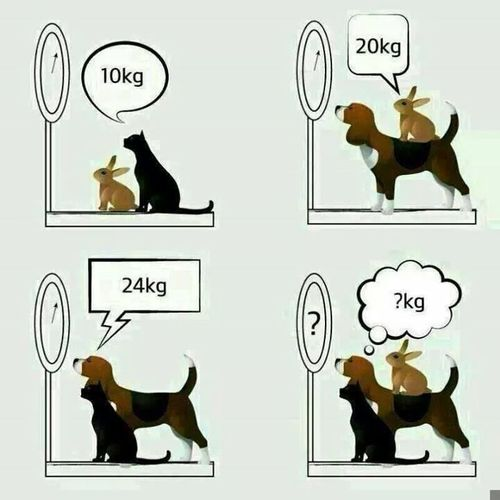Make the 10 coin pyramid on the left look like the inverted pyramid on the right by moving only 3 coins.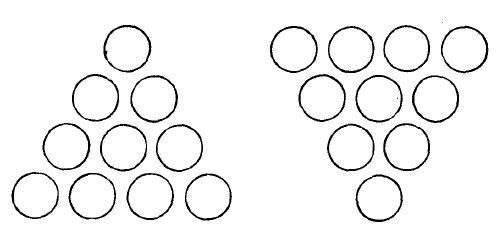How can you place the numbers 1 through 9 in a 3×3 grid such that every row, column, and the two diagonals all add up to 15?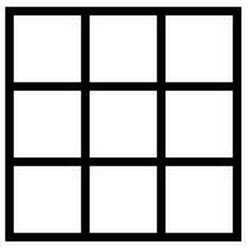Can you circle exactly four of these numbers such that the total is twelve?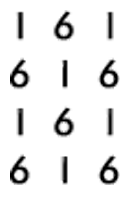There are 13 caves arranged in a circle. There is a thief hiding in one of the caves. Each day the thief can move to any one of the caves that is adjacent to the cave in which he was staying the previous day. And each day, two cops search any two caves of their choice for the thief. So, one cave is searched per cop per day.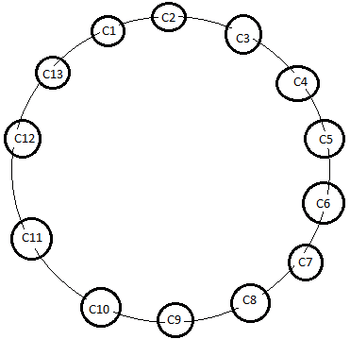The following conditions apply.

1. The thief may either move to an adjacent cave or stay in the same cave.
2. The cops can check any two caves each day, they do not need to be adjacent.
3. The thief only moves to the adjacent cave when the cops are making their rounds.
4. If the thief moves from cave X to adjacent cave Y and if one of the cops is going to check on either cave X or cave Y, the thief will be caught.

What is the minimum number of days to guarantee in which the cops can catch the thief?